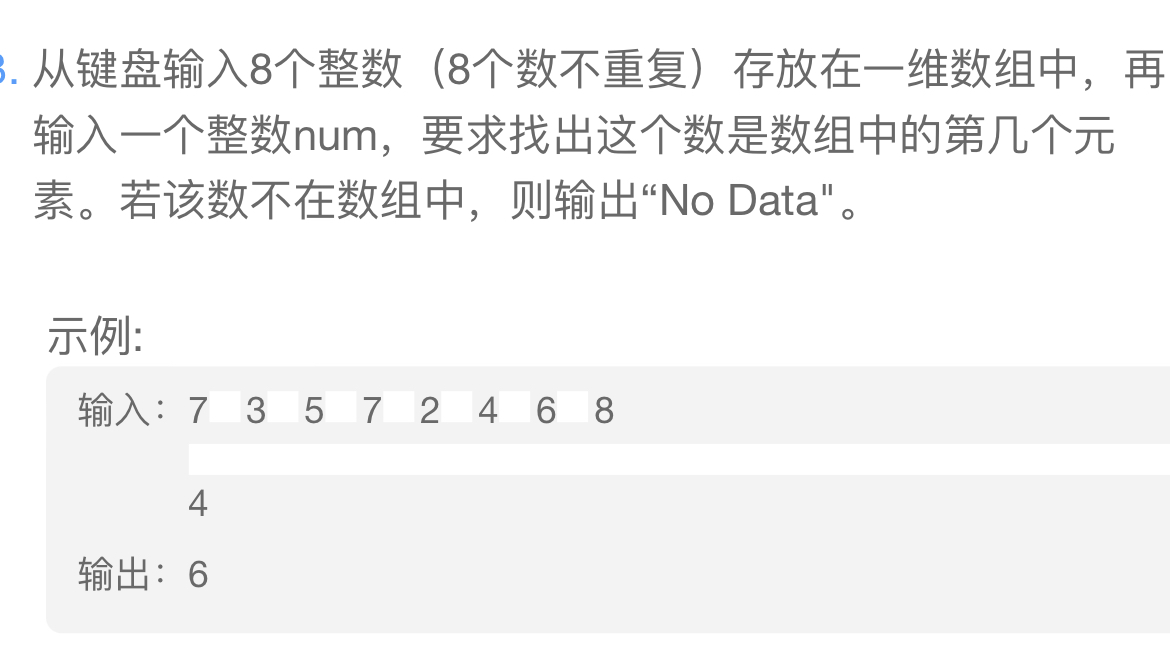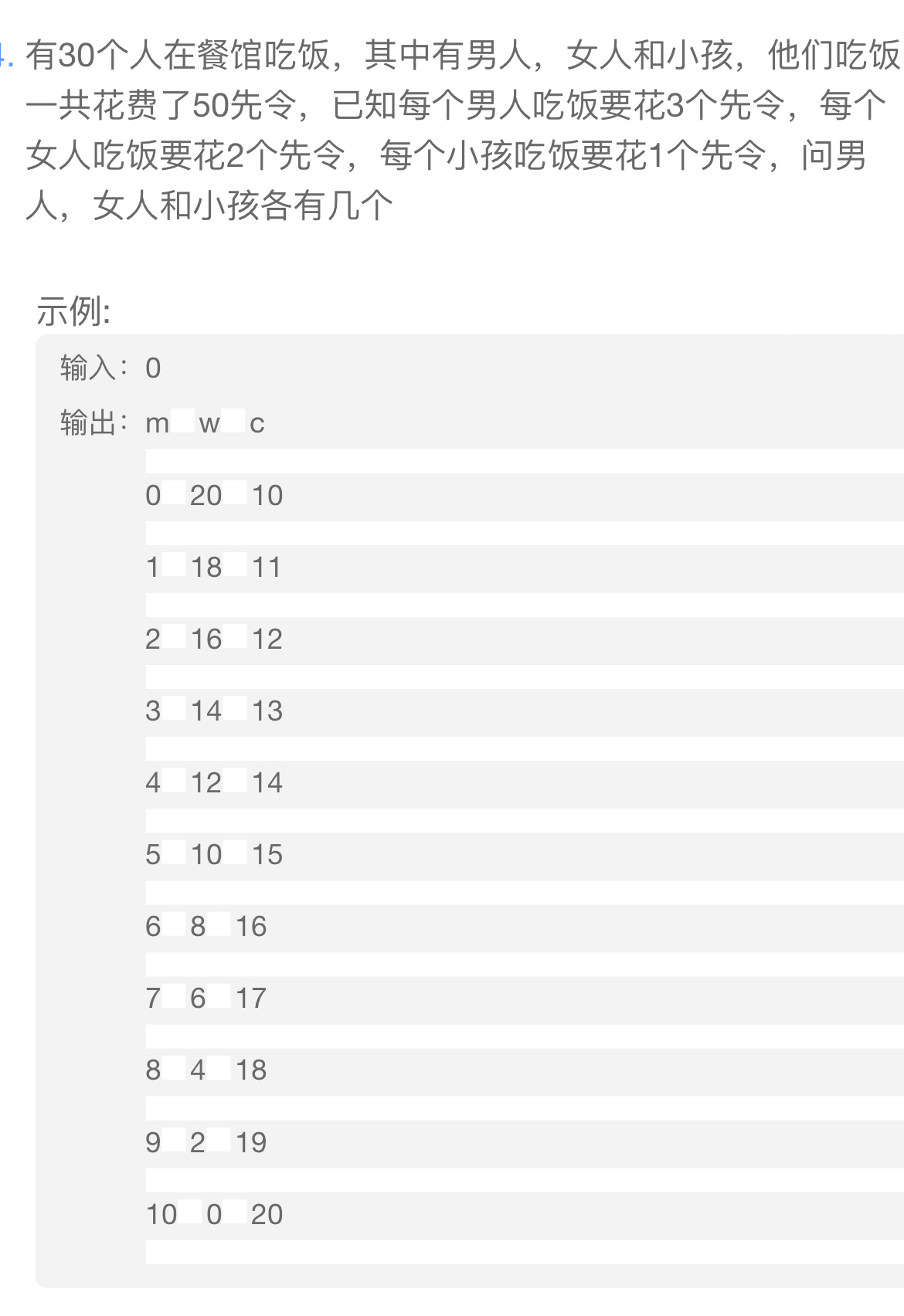2021-10-15 09:54

# 大一新生C++数组练习，在线求🥺• 好问题 提建议
• 收藏

#### 1条回答默认 最新

•qfl_sdu 2021-10-15 10:02
已采纳

1

``````#include <iostream>
using namespace std;
int main()
{
int i,a,num;
for(i=0;i<8;i++)
cin >> a[i];
cin >> num;
for(i=0;i<8;i++)
{
if(num == a[i])
break;
}
if(i<8)
cout << i+1;
else
cout << "No Data";
return 0;
}

``````

2

``````#include <iostream>
using namespace std;
int main()
{
int m=0,w=0,c = 0;
cout <<"m  w  c";
for (m=0;m<17;m++)
{
for(w=0;w<=25;w++)
{
c = 30 - m - w;
if( c>=0 && (3*m+2*w + c == 50) )
cout << endl <<m << "  " << w <<"  "<< c;
}
}
return 0;
}

``````
已采纳该答案
评论
解决 1 无用
打赏 举报